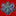## HEP Laboratory: Research Activities##\$B35MW(B

\$BK\8&5f<<\$G\$O!\$%S!<%`>WFM7?\$N9b%(%M%k%.!WFM!\$K\WFM%(%M%k%.!<\$G\$NAGN3;R

\$BAGN3;RJ*M}3X\$NBh0l\$NL\E*\$O!\$1'Ch\$r9=@.\$9\$kMWAG!JAGN3;R!K\$H\$=\$l\$i\$N4V\$KF/\$/Aj8_:nMQ\$NK\WFM@\\$+\$D>\:Y\$K8&5f\$9\$k\$3\$H(B, \$B\$^\$?\$=\$l\$i\$NN3;R\$KZ!\$%R%C%0%9N3;R\$NC5:w!\$I8=`LO7?\$rD6\$(\$k?7N3;R!&?78=>]\$NC5:w\$J\$I\$,5s\$2\$i\$l\$k!%(B

\$B\$^\$?!\$>-Mh\$NWFM7?2CB.4o(B Large Hadron Collider(LHC) \$B\$N(BATLAS\$B8!=P4o\$O!\$>WFM51EY\$r>e\$2\$?F3BN8!=P4o\$r3+H/\$7\$F\$\$\$k!%(B \$B\$5\$i\$K!\$7W2h!&@_7WCf\$NEE;R!&M[EE;R>WFM7?@~7A2CB.4o(B International Linear Collider(ILC) \$B\$KMQ\$\$\$i\$l\$k9b@-G=%+%m%j%a!<%?!JN3;R%(%M%k%.!@\B,Dj\$9\$k\$?\$a\$K\$O!\$>.\$5\$J

##\$B3X0LO@J8\$HF|K\J*M}3X2qH/I=(B